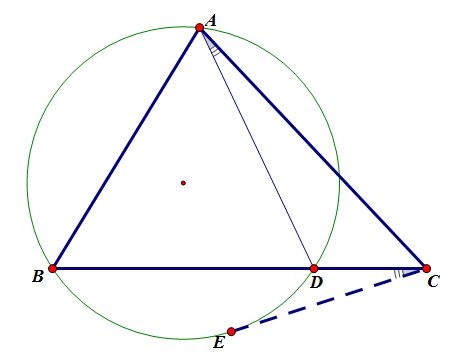# I Am Proud Of This Tangent

Geometry Level 5$D$ lies on segment $BC$, $CE$ is tangent to circumcircle $\odot ABD$ at $E$, and $\angle CAD=\angle BCE$.

If $\frac {5}{3}BC=AC+AB$ and $AC=33$. Find $CD$.

×